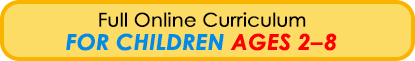Curriculum Overview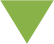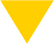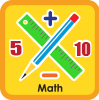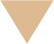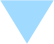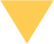Math

Mathematics is the language that we use to talk about quantities, shapes, and patterns.

Numbers
Shapes
Measurements
Patterns
Numbers

Recognizing numbers and knowing their names is an important first step in learning mathematics, but math education experts say that it’s also very important for children to develop number sense: the ability to relate number symbols (for example, 1, 2, and 3) to the amounts they stand for. Number sense includes these skills:
• Comparing quantities (more than and less than)
• Putting numbers in order
• Estimating quantities without counting
• Knowing when the answer to a math problem makes sense

Children should understand that the symbols 1, 2, 3, and so on stand for actual quantities of things and that mathematical statements, like 2 + 3 = 5 or 6 - 2 = 4, are ways of describing how those quantities relate to each other. In fact, many math education experts recommend that children first learn the concepts of addition and subtraction by combining or separating actual groups of objects (or pictures of objects), rather than by working with the symbols for plus (+) and minus (-).

In addition to understanding the importance of number sense, math educators must also recognize that when children are learning the meanings of the number symbols 1, 2, 3, and so on, they are actually learning several different ideas at the same time. For example, to understand the meaning of the symbol 2, a child would need to grasp all of these facts:
• The symbol 2 stands for the spoken word “two”.
• The symbol 2 can stand for a certain number of objects.
• The symbol 2 can stand for the second position in a sequence.
• When counting, 2 comes after 1 and before 3.
• The written word two also stands for the spoken word “two”, so 2 and two mean the same thing.

ABCmouse.com’s Math curriculum includes many different ways that children can learn about numbers and develop number sense.“Like” us on FacebookFollow us on TwitterFollow us on PinterestEditor’s Choice
AwardParents’ Choice
GOLD AwardTeachers’ Choice
AwardMom’s Choice
Awards GOLDSPECIAL OFFER
Get Your First Month FREE!I agree to receive emails from ABCmouse.comSubmit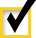Click Here to
LEARN MORE
SUBSCRIPTION
PRICING
\$995
per month